Courses

# The Root Locus Technique - 1

## 10 Questions MCQ Test Topicwise Question Bank for Electronics Engineering | The Root Locus Technique - 1

Description
This mock test of The Root Locus Technique - 1 for Electrical Engineering (EE) helps you for every Electrical Engineering (EE) entrance exam. This contains 10 Multiple Choice Questions for Electrical Engineering (EE) The Root Locus Technique - 1 (mcq) to study with solutions a complete question bank. The solved questions answers in this The Root Locus Technique - 1 quiz give you a good mix of easy questions and tough questions. Electrical Engineering (EE) students definitely take this The Root Locus Technique - 1 exercise for a better result in the exam. You can find other The Root Locus Technique - 1 extra questions, long questions & short questions for Electrical Engineering (EE) on EduRev as well by searching above.
QUESTION: 1

### Which of the following option is correct? The root locus is the path of the roots of the characteristic equation traced out in the s-plane

Solution:

The root locus is defined as location of closed loop poles when system gain k is varied from zero to infinity.

QUESTION: 2

### Number of intersection of the asymptotes of the complete root loci is

Solution:

Centroid is the intersection point of asymptotes of the complete root loci which lie on the real axis.

QUESTION: 3

### The open-loop transfer function of a unity feedback control system is given by: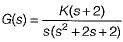The centroid and the angle of root locus asymptotes are respectively

Solution: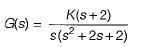Number of open loop poies = P = 3
and number of open loop zeros = Z = 1
Poles are at: s = -1 ± j, 0 and zero is at s = -2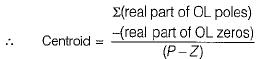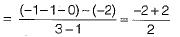= 0
Here, P - Z = 2
So, angle of asymptotes are given by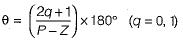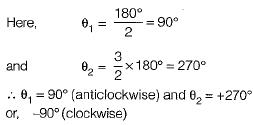QUESTION: 4

Consider the loop transfer function K(s + 6) / (s + 3)(s +5 ) In the root locus diagram the centroid will be located at:

Solution:

Centroid = Sum of real part of open loop pole-sum of real part of open loop zeros / P - Z.

QUESTION: 5

Assertion (A): Root locus is a graphical method in which roots of the characteristic equation are plotted in s-plane for the different value of parameter.
Reason (R): The locus of the roots of the characteristic equation when gain is varied from zero to infinity is called root locus.

Solution:
QUESTION: 6

Assertion ( A ) : An addition of real pole at s = - p0 in the transfer function G(s)H(s) of a control system results in the increase of stability margin.
Reason ( R ) : An addition of real pole at s = - p0 in the transfer function G(s)H(s) will make the resultant root loci bend towards the right.

Solution:

Addition of real pole to an open loop T.F. decreases the stability because root locus shifts towards right of s-plane. Hence, assertion is a false statement.

QUESTION: 7

The open-loop transfer function of a closed loop control system is given as: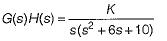Which of the following statements is correct about the root locus of the above system?

Solution: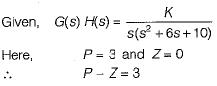Number of branches terminating at infinity = 3 = P - Z.
Number of branches terminating at zero = Z= 0.
Poles are at s = 0 and s = -3 ± j1.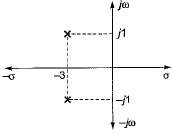Since, three number of poles have to terminate at infinity, therefore the two complex poles will terminate at infinity due to which there will be intersection of root locus branches with jω-axis. Now, characteristic equation is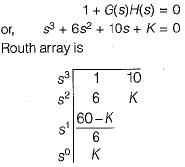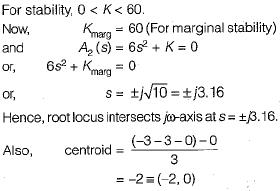QUESTION: 8

The root locus of s(s - 1) + K(s + 1) = 0 is a circle. The co-ordinates of the centre and the radius (in units) of this circle are respectively

Solution:

Given, s(s - 1) + K(s + 1) = 0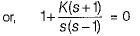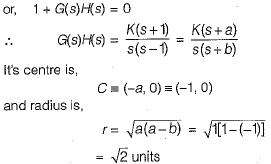QUESTION: 9

What is the open-loop transfer function of a unity feedback control system having root locus shown in the following figure?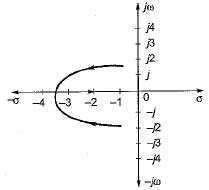Solution:

Branches of root locus either terminate at infinity or zero. Here, one branch is terminating at s = -2, therefore there is a zero at s -2. Since two branches are meeting between s = -3 and s = -4, therefore there is a breakaway point between s = -3 and -4, Hence, there must be poles at s = (-1 ± j).
Therefore, open loop T.F. is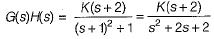Here, P = 2 and Z = 1.
Thus, one branch terminates at s = -2 (zero) and one branch terminate sat infinity ( P - Z = 1).

QUESTION: 10

A control system has G(s)H(s)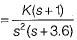(0 < K < ∞). The number of breakaway point in the root locus diagram is/are

Solution: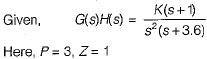∴  Number of branches terminating at infinity = P - Z = 2.
Number of branches terminating at zero = Z = 1.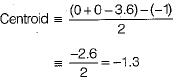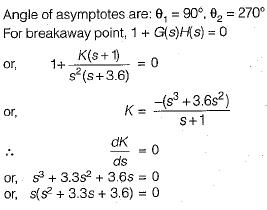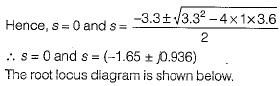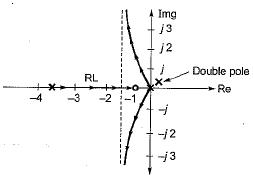Here, (-1.65 ± j0.936) is not a part of root locus. Thus, it has only one breakaway point s = 0.